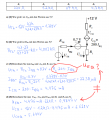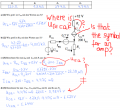# Emitter circuit / equivalent circuit / calculate voltages.

#### Tobias Hildebrandt

Joined Jan 1, 2016
44Hello,
I have a problem with part D of this question. In part D our Prof wants to know U(EQ), U(CQ) and U(ECQ) using the values for R1 and R2 from the table above.
In question C we calculated I(BQ) and I(CQ) and I was under the impression that we calculated U(EQ) too. Isn't U(EQ) = R1 * 201 (!!!) * I(BQ) and not I(CQ) * R1???
My next problem is: U(CQ) = R2 * I(CQ) and U(ECQ) = 12 V - U(CQ) - U(EQ). This is wrong(!), but why?

Tobias

#### WBahn

Joined Mar 31, 2012
29,179
For your second question, isn't it I(EQ) that is flowing through R1 and not I(CQ)?

What is I(EQ) in terms of I(BQ) and (CQ)?

What is I(CQ) in terms of I(BQ) and beta?

What is I(EQ) in terms of I(BQ) and beta?

How does that compare to 201*I(BQ)?

#### Tobias Hildebrandt

Joined Jan 1, 2016
44
-What is I(EQ) in terms of I(BQ) and (CQ)?

If you mean (CQ), than I have no idea. Type or do I miss sth.? If you meant in terms of I(BQ) and I(CQ), than my answer is: I(EQ) = I(BQ) + I(CQ) ; I(CQ) = 200 * I(BQ) -> I(EQ) = I(BQ) + 200 * I(BQ) = 201 * I(BQ).

That is what you wanted to know, aye?

BUT if this is the case, than the answer provided by our Prof is wrong. Correct?

#### Tobias Hildebrandt

Joined Jan 1, 2016
44Couple of questions!
1) If a current runs through a resistor forexample, the voltage is across two points. Where is U(CQ) and U(ECQ) on this circuit? Can I even see it on the equivalent circuit or can you only see it on a circuit that includes the transistor?
2) This is a really stupid question and I really should know that, but what is that symbol? I marked it on the pic above.

Cheers
Tobias

#### Jony130

Joined Feb 17, 2009
5,457
•Tobias Hildebrandt

#### MrAl

Joined Jun 17, 2014
10,620
View attachment 99074
Hello,
I have a problem with part D of this question. In part D our Prof wants to know U(EQ), U(CQ) and U(ECQ) using the values for R1 and R2 from the table above.
In question C we calculated I(BQ) and I(CQ) and I was under the impression that we calculated U(EQ) too. Isn't U(EQ) = R1 * 201 (!!!) * I(BQ) and not I(CQ) * R1???
My next problem is: U(CQ) = R2 * I(CQ) and U(ECQ) = 12 V - U(CQ) - U(EQ). This is wrong(!), but why?

Tobias
Hi,

You seem to have a point here. It is written as 201*Ibq but he actually calculated 200*Ibq. That means there is a small difference.
Perhaps it was an oversight, or alternately he wanted you to know both techniques.

The right current through R1 is (B+1)*Ibq, not just (B)*Ibq, and so the current through R1 will be slightly higher than written:
4.498ma

and so the voltage across R1 will be slightly higher than written:
0.9896v

Using the collector current instead of the true emitter current is not a rare technique and is done very often as an estimate of the emitter current because the base current is often very insignificant and the gain of the transistor is not known exactly in real life anyway, so any error introduced by using the collector current instead of the emitter current is swamped by the usual error in not knowing the exact Beta.
With this problem however it appears that he *was* using the correct current, the emitter current, to show the method of calculation, but then resorted to the more often used version when he actually calculated the actual current and therefore the actual voltage.
This can only be resolved by asking the instructor what he really wanted, because maybe he wanted you to be aware of the exact calculation yet at the same time be aware of the commonly used estimation technique. Alternately he just made a simple mistake.
This is if i understand your question correctly, but following your attachment text work that's how it appears to be.

•Tobias Hildebrandt